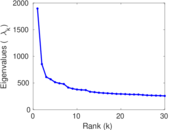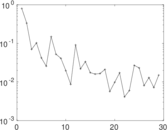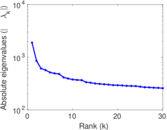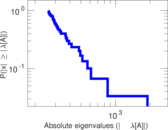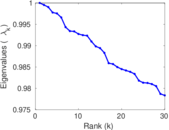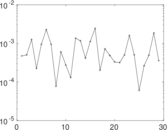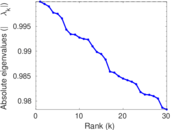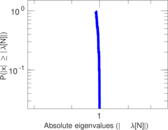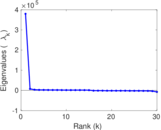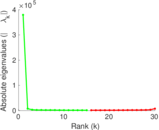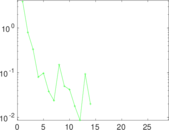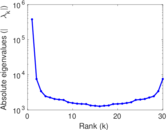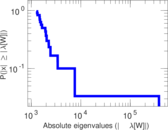# Linux kernel mailing list threads

This bipartite network contains contributions of persons to various threads of the Linux kernel mailing list. An edge represents a post; left nodes are persons and right nodes represent threads in the mailing list.

 Code `LK` Internal name `lkml_person-thread` Name Linux kernel mailing list threads AvailabilityDataset is available for download Consistency checkDataset passed all tests Category Interaction network Dataset timestamp 2006 ⋯ 2013 Node meaning Person, thread Edge meaning Post Network formatBipartite, undirected Edge typeUnweighted, multiple edges Temporal dataEdges are annotated with timestamps

## Statistics

 Size n = 379,554 Left size n1 = 42,045 Right size n2 = 337,509 Volume m = 1,565,683 Unique edge count m̿ = 599,858 Wedge count s = 1,002,436,389 Square count q = 11,033,932 4-Tour count T4 = 4,099,266,604 Maximum degree dmax = 46,620 Maximum left degree d1max = 46,620 Maximum right degree d2max = 9,101 Average degree d = 8.250 12 Average left degree d1 = 37.238 3 Average right degree d2 = 4.638 94 Fill p = 4.227 16 × 10−5 Average edge multiplicity m̃ = 2.610 09 Size of LCC N = 364,645 Diameter δ = 22 50-Percentile effective diameter δ0.5 = 3.791 92 90-Percentile effective diameter δ0.9 = 5.656 67 Median distance δM = 4 Mean distance δm = 4.609 19 Gini coefficient G = 0.792 924 Balanced inequality ratio P = 0.182 741 Left balanced inequality ratio P1 = 0.067 359 1 Right balanced inequality ratio P2 = 0.252 499 Relative edge distribution entropy Her = 0.808 470 Power law exponent γ = 3.711 04 Tail power law exponent γt = 1.751 00 Degree assortativity ρ = −0.079 021 3 Degree assortativity p-value pρ = 0.000 00 Spectral norm α = 1,895.58 Algebraic connectivity a = 0.002 041 70 Controllability C = 340,372 Relative controllability Cr = 0.896 768

## Plots

### Degree distribution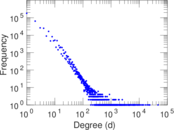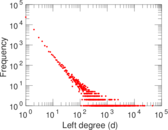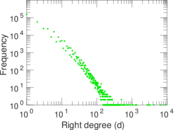### Cumulative degree distribution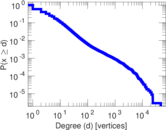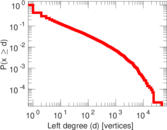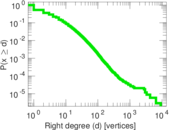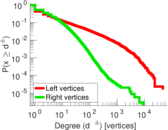### Lorenz curve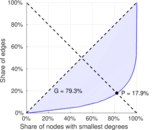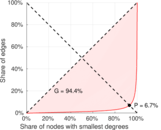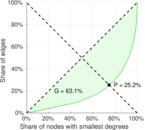### Spectral distribution of the adjacency matrix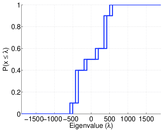### Spectral distribution of the normalized adjacency matrix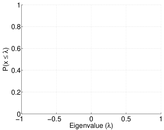### Spectral distribution of the Laplacian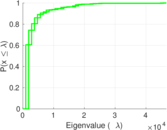### Spectral graph drawing based on the adjacency matrix### Spectral graph drawing based on the Laplacian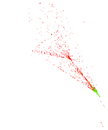### Spectral graph drawing based on the normalized adjacency matrix### Degree assortativity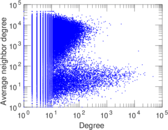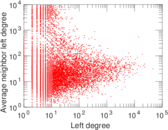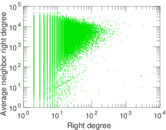### Zipf plot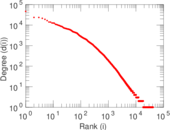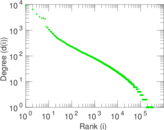### Hop distribution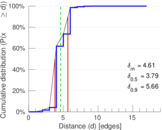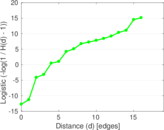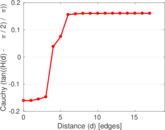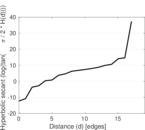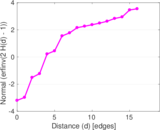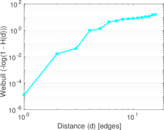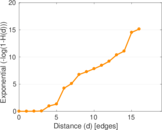### Edge weight/multiplicity distribution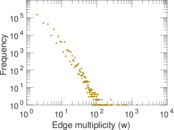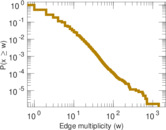### Temporal distribution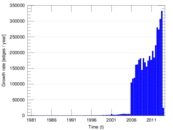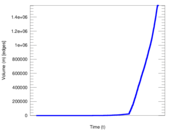### Diameter/density evolution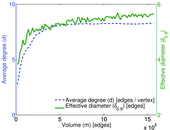### Inter-event distribution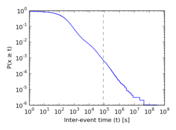### Node-level inter-event distribution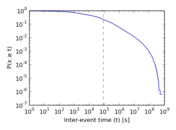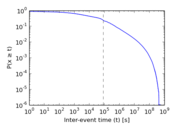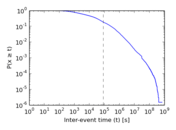### Matrix decompositions plots